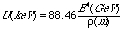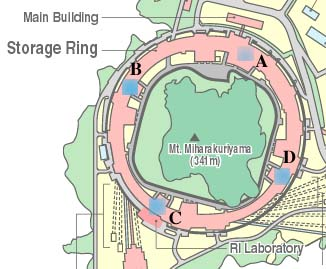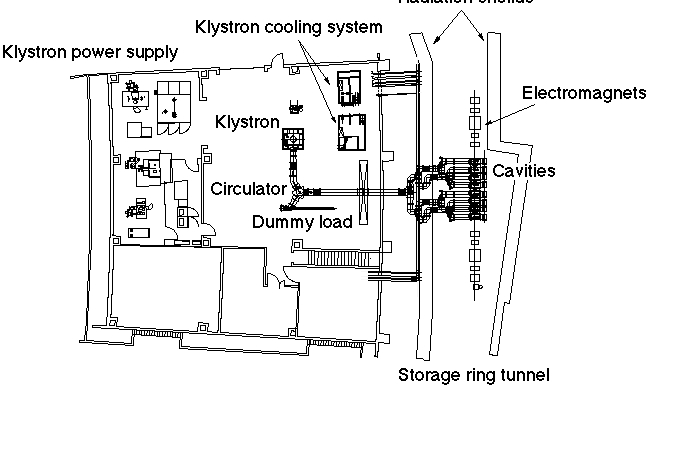## RF acceleration system

The aim of the RF system is
to compensate for the energy loss of the electron beam,
to keep the longitudinal stability,
and to determine the radius of the ring.

The energy loss per turn U is expressed aswhere E is the energy of the beam in unit of GeV, r is the bending radium of dipole magnet.
At the SPring-8 E is 8GeV and r is 39m, so U becomes 9MeV.
If the stored current is 100mA, the power consumed by the beam is 900kW (225kW/station).
In the actual operation The loss is increased by the loss by Insertion devices and so on.

There are four RF stations distributed along the ring.
Each station has eight accelerating cavities.

### RF related topics

• phase adjustment of four stations
• voltage calibration
• reduction of coherent synchrotron oscillation
• cavity conditioning
• troubles

## librarylocation of four RF stations A,B,C and Dplan of RF station